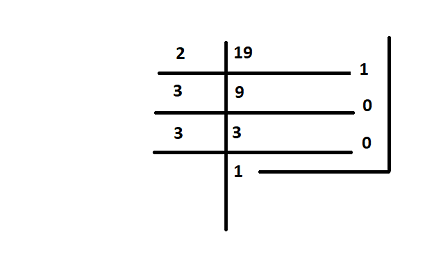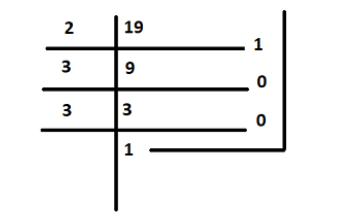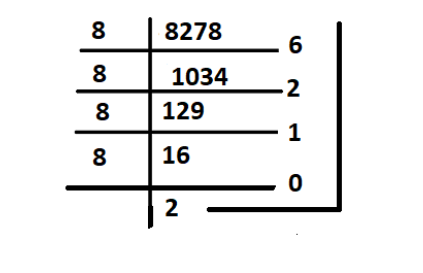# What is a number system?

• Last Updated : 03 Sep, 2021

The arithmetic value which is used for representing the quantity and used in making calculations are defined as NUMBERS. A symbol like “4, 5, 6” which represents a number is known as numerals. Without numbers, counting things is not possible, date, time, money, etc. these numbers are also used for measurement and used for labelling. The properties of numbers make them helpful in performing arithmetic operations on them.  These numbers can be written in numeric forms and also in words.

For example, 3 is written as three in words, 35 is written as thirty-five in words, etc. Students can write the numbers from 1 to 100 in words to learn more. There are different types of numbers, which we can learn. They are whole and natural numbers, odd and even numbers, rational and irrational numbers, etc.

Attention reader! All those who say programming isn't for kids, just haven't met the right mentors yet. Join the  Demo Class for First Step to Coding Coursespecifically designed for students of class 8 to 12.

The students will get to learn more about the world of programming in these free classes which will definitely help them in making a wise career choice in the future.

### Number and Its Types

Numbers used in mathematics are mostly decimal number systems. In the decimal number system, digits used are from 0 to 9 and base 10 is used. There are many types of numbers in decimal number system, below are some of the types of numbers mentioned,

• Numbers that are represented on the right side of the zero are termed Positive Numbers. The value of these numbers increases on moving towards the right. Positive numbers are used for Addition between numbers. Example: 1, 2, 3, 4.
• Numbers that are represented on the left side of the zero are termed Negative Numbers. The value of these numbers decreases on moving towards the left. Negative numbers are used for Subtraction between numbers. Example: -1, -2, -3, -4.
• Natural Numbers are the most basic type of Numbers that range from 1 to infinity. These numbers are also called Positive Numbers or Counting Numbers. Natural Numbers are represented by the symbol N.
• Whole Numbers are basically the Natural Numbers, but they also include ‘zero’. Whole numbers are represented by the symbol W.
• Integers are the collection of Whole Numbers plus the negative values of the Natural Numbers. Integers do not include fraction numbers i.e. they can’t be written in a/b form. The range of Integers is from the Infinity at the Negative end and Infinity at the Positive end, including zero. Integers are represented by the symbol Z.
• Rational numbers are the numbers that can be represented in the fraction form i.e. a/b. Here, a and b both are integers and b≠0. All the fractions are rational numbers but not all the rational numbers are fractions.
• Irrational numbers are the numbers that can’t be represented in the form of fractions i.e. they can not be written as a/b.
• Numbers that do not have any factors other than 1 and the number itself are termed as Prime Numbers. All the numbers other than Prime Numbers are termed as Composite Numbers except 0. Zero is neither prime nor a composite number.

### What is a number system?

A Number system is a method of showing numbers by writing, which is a mathematical way of representing the numbers of a given set, by using the numbers or symbols in a mathematical manner. The writing system for denoting numbers using digits or symbols in a logical manner is defined as a Number system. The numeral system Represents a useful set of numbers, reflects the arithmetic and algebraic structure of a number, and Provides standard representation. The digits from 0 to 9 can be used to form all the numbers. With these digits, anyone can create infinite numbers. For example, 156,3907, 3456, 1298, 784859 etc.

### Types of Number Systems

Based on the base value and the number of allowed digits, number systems are of many types. The four common types of Number System are:

• Decimal Number System
• Binary Number System
• Octal Number System

Decimal Number System

Number system with a base value of 10 is termed a Decimal number system. It uses 10 digits i.e. 0-9 for the creation of numbers. Here, each digit in the number is at a specific place with place value a product of different powers of 10. Here, the place value is termed from right to left as first place value called units, second to the left as Tens, so on Hundreds, Thousands, etc. Here, units have the place value as 100, tens have the place value as 101, hundreds as 102, thousands as 103, and so on.

For example, 10264 has place values as,

(1 × 104) + (0 × 103) + (2 × 102) + (6 × 101) + (4 × 100)

= 1 × 10000 + 0 × 1000 + 2 × 100 + 6 × 10 + 4 × 1

= 10000 + 0 + 200 + 60 + 4

= 10264

Binary Number System

Number System with base value 2 is termed as Binary number system. It uses 2 digits i.e. 0 and 1 for the creation of numbers. The numbers formed using these two digits are termed Binary Numbers. The binary number system is very useful in electronic devices and computer systems because it can be easily performed using just two states ON and OFF i.e. 0 and 1.

Decimal Numbers 0-9 are represented in binary as: 0, 1, 10, 11, 100, 101, 110, 111, 1000, and 1001

For example, 14 can be written as 1110, 19 can be written as 10011, 50 can be written as 110010.

Example of 19 in the binary systemHere 19 can be written as 10011

Octal Number System

Octal Number System is one in which the base value is 8. It uses 8 digits i.e. 0-7 for the creation of Octal Numbers. Octal Numbers can be converted to Decimal values by multiplying each digit with the place value and then adding the result. Here the place values are 80, 81, and 82. Octal Numbers are useful for the representation of UTF8 Numbers. Example,

(135)10 can be written as (207)8

(215)10 can be written as (327)8

Number System with base value 16 is termed as Hexadecimal Number System. It uses 16 digits for the creation of its numbers. Digits from 0-9 are taken like the digits in the decimal number system but the digits from 10-15 are represented as A-F i.e. 10 is represented as A, 11 as B, 12 as C, 13 as D, 14 as E, and 15 as F. Hexadecimal Numbers are useful for handling memory address locations. Examples,

(255)10  can be written as (FF)16

(1096)10  can be written as (448)16

(4090)10  can be written as (FFA)16

### Sample Problems

Question 1: Convert (18)10 as a binary number?

Solution:Therefore (18)10 = (1001)2

Question 2: Convert 3258 into a decimal?

Solution:

325 = 3 × 82 + 2 × 81 + 5 × 8

= 3 × 64 + 2 × 8 + 5 × 1

= 192 + 16 + 5

= 21310

Question 3: Convert (2056)16 into an octal number?

Solution:

Here (2056)16 is in hexadecimal form

First we will convert into decimal form from hexadecimal.

(2056)16 = 2 × 163 + 0 × 162 + 5 × 161 + 6 × 160

= 2 × 4096 + 0 + 80 + 6

= 8192 + 0 + 80 + 6

= (8278)10

Now convert this decimal number into octal number by dividing it by 8So will take the value of remainder from 20126

(8278)10 = ( 20126)8

Therefore, ( 2056 )16 = ( 20126 )8

Question 4: Convert (101110)2 into octal number.

Solution:

Given (101110)2 a binary number, to convert it into octal number

Using the above table we can write given number as,

101 110 i,e.

101 = 5

110 = 6

So (101110)2 in octal number is (56)8

My Personal Notes arrow_drop_up